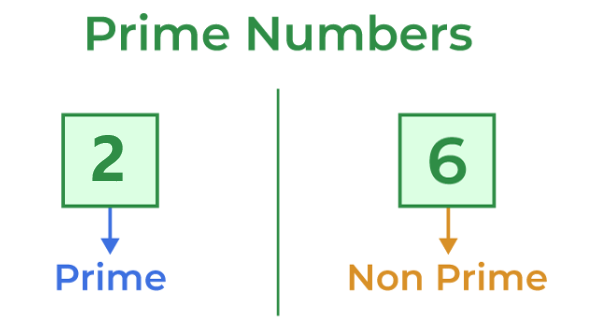Open In App

# C++ Program to check Prime Number

A prime is a natural number greater than 1 that has no positive divisors other than 1 and itself. For example, 5 is prime but 10 is not prime. In this article, we will learn how to write a C++ program to check whether a number is prime or not.## Algorithm to Check Prime Numbers in C++

• Run a loop from 2 to n/2 using a variable i.
• Inside the loop, check whether n is divisible by i using the modulo operator ( n % i == 0 ).
• If n is not divisible by i, it means n is a prime number.
• If n is divisible by i, it means n has divisors other than 1 and itself, and thus, it is not a prime number.

## Prime Number Program in C++

Below is the C++ program to check if a number is a prime number or not.

## C++

 `// A school method based C++ program``// to check if a number is prime``#include ``using` `namespace` `std;` `bool` `isPrime(``int` `n)``{``    ``// Corner case``    ``if` `(n <= 1)``        ``return` `false``;` `    ``// Check from 2 to n-1``    ``for` `(``int` `i = 2; i <= n / 2; i++)``        ``if` `(n % i == 0)``            ``return` `false``;` `    ``return` `true``;``}` `// Driver code``int` `main()``{``    ``isPrime(11) ? cout << ``" true\n"` `: cout << ``" false\n"``;``    ``isPrime(15) ? cout << ``" true\n"` `: cout << ``" false\n"``;``    ``return` `0;``}`

Output

``` true
false

```

### Complexity Analysis

• Time Complexity: O(n)
• Auxiliary Space: O(1)